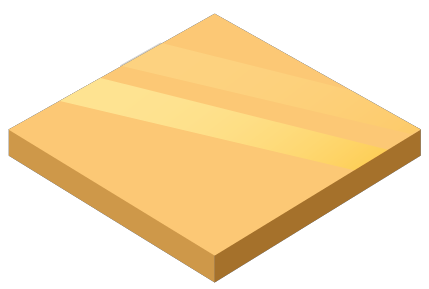# Gold Weight Calculator

Created by Luis Hoyos
Last updated: Nov 05, 2022

If you're carrying out a DIY project, this gold-weight calculator can be a great ally. The idea behind this calculator is to help people who have a block of metal of some shape and want to melt it and make something, considering the wastage occasioned in the process.

You can use this tool for various purposes, such as:

• Calculate the weight of the gold or silver shape;
• Determine the total cost of the raw metal block necessary to make the new shape; and
• Use this calculator as a gold converter between the different finenesses, comparing the different amounts of material and prices required to make a shape with various materials.

🙋 If you already know the volume of your gold or silver metal block, you can simply input it without having to type in the dimensions.

## Material fineness

Metal fineness is a measure of the purity of gold and silver. We express it as a percentage of the total weight of the metal composed of pure gold or silver. For example, a gold alloy with a fineness of 900 would contain 900 parts per thousand of pure gold. The remainder of the alloy would contain other metals, such as copper or silver.

To be considered legal tender, gold and silver coins must have a minimum fineness of 0.900. However, many coins have much higher ones, such as 0.999 (fine gold) or 0.9999 (ultra-fine gold).

## How to calculate gold weight?

For any shape, this tool uses the following formula to calculate the gold weight:

$\footnotesize \mathrm {Mass\!\! =\!\! Volume\!\! \times \!\!Density\!\! \times\!\! (1\!\! -\!\! Wastage)}$

, where:

• $\text {Mass}$ — Mass of the object to fabricate;
• $\text {Volume}$ — Volume of the gold/silver object we'll melt to create the new shape;
• $\text {Density}$ — Density of the fineness or material used; and
• $\text {Wastage}$Percentage of the melted object wasted in the process.

🙋 To calculate the mass in pounds (lb), we must input the volume in cubic feet (ft³) and the density in lb/ft³. To get it in kilograms (kg), the volume must be in cubic meters () and the density in kg/m³.

The previous formula is the same one used in our density calculator, with the difference that now we consider the material wasted.

The density is a property that represents the silver/gold weight by volume unit. It will depend on the fineness of the material, and you can use the calculator as a gold converter and look at the corresponding density of the different finenesses.

We calculate the volume of the gold and silver shapes with the following formulas:

$\footnotesize \mathrm{Sheet\ \! volume\!\! =\!\! Length\!\! \times \!\!W\!idth\!\! \times \!\!Thickness} \\ \mathrm{Wire\ volume} = \pi \times r^2 \times L \\ \mathrm{Rondelle\ volume} = \pi \times r^2 \times T \\ \mathrm{Tube\ volume} = \pi \times (r_2^2 - r_1^2) \times L$

, where:

• $r$ — Wire or rondelle radius;
• $L$ — Wire or tube length; and
• $r_1$ and $r_2$ — Tube inner and outer radius, respectively.

For the sheet, we use the same formula used in the volume of a rectangular prism calculator. For the wire and rondelle, we use the cylinder volume formula (length and thickness equivalent quantities), while for the tube, we use the equation for a hollow cylinder. You can learn more about those formulas in our cylinder volume calculator.

## How to calculate the gold cost?

The cost of the material is simply the mass times the cost per unit mass, but without subtracting the material wasted, as we must pay for it anyway:

$\footnotesize \mathrm{Cost = Mass \times Cost\ per\ unit\ mass}$
$\footnotesize \mathrm {Mass = Volume \times Density}$

The $\text {Mass}$ and the $\text {Cost per unit mass}$ must use the same mass units. For example, if we express the cost per mass as dollars per ounce, the mass must also be in ounces.

Luis Hoyos
Shape
SheetMaterial
Material (fineness)
Gold - 333
Density
lb/cu ft
Wastage
%
Sheet
Length
ft
Width
ft
Thickness
in
Volume
cu ft
Result
Mass
lb
Cost per unit mass
$/ oz t Cost$
People also viewed…

### Brick

Use this brick calculator to find out the number of bricks you need for any brick wall size. The number is calculated to the nearest whole number without taking into account any need to cut bricks. The unit menus can be used to choose appropriate units.

### Loan calculator

Our loan calculator will quickly tell you everything you need to know about your loans!